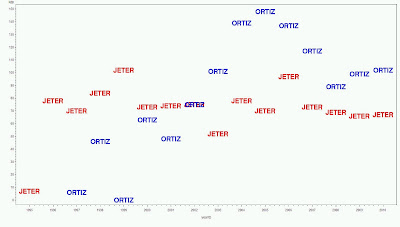## Tuesday, April 5, 2011

### Example 8.33: Merging data sets one-to-manyIt's often necessary to combine data from two data sets for further analysis. Such merging can be one-to-one, many-to-one, and many-to-many. The most common form is the one-to-one match, which we cover in section 1.5.7. Today we look at a one-to-many merge.

Since the Major League baseball season started last Thursday, we'll use baseball as an example. Sean Lahman has compiled a large set of baseball data. In fact, it's large enough that it's only hosted in zipped form. The zipped comma-delimited files, with data through the 2010 season, can be downloaded here.

One file you get is the batting.csv file. This contains yearly batting data for all players. However, if you want to identify players by name, you have to use the playerid variable to link to the master.csv data set, which has names and other information. We'll add the names to each row of the batting data set. Then we can pull up players' batting data directly by name instead of with the playerid

SAS

We'll start by reading the data from the csv files using proc import (section 1.1.4).
`proc import datafile="c:\ken\baseball\master.csv"   out=bbmaster dbms=dlm;  delimiter=',';  getnames=yes;run;proc import datafile="c:\ken\baseball\batting.csv"   out=bbbatting dbms=dlm;  delimiter=',';  getnames=yes;run;`

Then we sort on playerid in both data sets and use the merge and by statements to link them (section 1.5.7). SAS replicates each row of the master data set for every row of the batting data set with the matching by values.
`proc sort data=bbmaster; by playerid; run;proc sort data=bbbatting; by playerid; run;data bbboth; merge bbmaster bbbatting;  by playerid;run;`

Then we can use the data! Here, we plot the annual (regular season) RBIs of Derek Jeter and David Ortiz. Note the use of the v= option to the symbol statement (section 5.2.2) to display the players' names at the plot locations, and the offset option in the axis definition to make extra space for those names to plot.
`symbol1 i=none font=swissb v="JETER" h=2 c=red;symbol2 i=none font=swissb v="ORTIZ" h=2 c=blue;axis1 offset = (1.5cm,1.5cm);axis2 offset = (.5cm,);proc gplot data=bbboth;where (namelast="Jeter" and namefirst="Derek") or       (namelast="Ortiz" and namefirst="David");plot rbi * yearid = namelast / haxis=axis1 vaxis=axis2 nolegend;run;`

The result is shown above.

R
We start here by reading the data sets. Then we use the merge() function to generate the desired dataset. As with SAS, the default behavior of the merging facility does what we need in this case.
`master = read.csv("bball/Master.csv")batting = read.csv("bball/Batting.csv")mergebb = merge(batting,master)`

To make the plot, we first make a new data set with just the information about Jeter and Ortiz. This isn't really necessary, but it does make typing slightly less awkward in later steps. Then we make an empty plot. In order to make room for the names (which are much bigger than usual plot symbols) we have to set the x and y limits manually (section 5.3.7).
`jo = mergebb[  (mergebb\$nameLast == "Jeter" & mergebb\$nameFirst == "Derek") |   (mergebb\$nameLast == "Ortiz" & mergebb\$nameFirst == "David"),]plot(jo\$RBI~jo\$yearID, type = "n",xlim = c(1993, 2012),   ylim = c(-10,160), xlab = "Year", ylab = "RBI")`

Then we can add the text values, using the text() function (section 5.2.11). We do this by separately pulling rows from the new jo dataset. In the reduced data set, we can specify rows using last names only.
`text(jo\$yearID[jo\$nameLast == "Jeter"], jo\$RBI[jo\$nameLast == "Jeter"],  "JETER", col="red")text(jo\$yearID[jo\$nameLast == "Ortiz"], jo\$RBI[jo\$nameLast == "Ortiz"],  "ORTIZ", col = "blue")`

The result is seen below. David Ortiz has driven in more runs than Derek Jeter since about 2002 (Go Sox!).

Note that if space requirements prevent making a single massive dataset with many replicated rows, you can generate a lookup vector using the match() function, and use this to make the short data set. This version won't have the names in it, though.
`matchlist = match(batting\$playerID, master\$playerID)lastname.batting = master\$nameLast[matchlist]firstname.batting = master\$nameFirst[matchlist]jo2 = batting[  (lastname.batting == "Jeter" & firstname.batting == "Derek") |   (lastname.batting == "Ortiz" & firstname.batting == "David"),]`